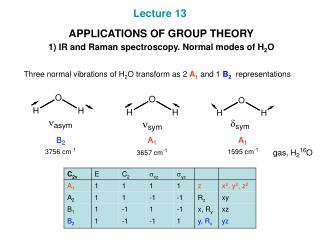Download PresentationLecture 13 APPLICATIONS OF GROUP THEORY 1) IR and Raman spectroscopy. Normal modes of H 2 O

# Lecture 13 APPLICATIONS OF GROUP THEORY 1) IR and Raman spectroscopy. Normal modes of H 2 O - PowerPoint PPT PresentationDownload Presentation## Lecture 13 APPLICATIONS OF GROUP THEORY 1) IR and Raman spectroscopy. Normal modes of H 2 O

- - - - - - - - - - - - - - - - - - - - - - - - - - - E N D - - - - - - - - - - - - - - - - - - - - - - - - - - -
##### Presentation Transcript

1. Lecture 13 APPLICATIONS OF GROUP THEORY1) IR and Raman spectroscopy. Normal modes of H2O Three normal vibrations of H2O transform as 2 A1 and 1 B2 representations

2. 2) Selection rules for IR and Raman active bands • A normal vibration is IR active if it transforms as x, y, z or any of their linear combinations. • A normal vibration is Raman active if it transforms as quadratic functions x2, y2, z2, xy, xz or yz.

3. 3) Selected vibrational modes • For polyatomic species considering full set of normal modes is usually very time consuming. • Consideration of only selected vibrational modes is simpler and often very important. The modes may be, for example, only stretching modes of a particular type. • Combined with group theoretical analysis, vibration spectroscopy may allow for distinction of isomers. • In the simplest cases the isomers can be distinguished by the number of stretching bands observed for particular bonds in IR spectra of these isomers (see the example below). • Let us see, how group theory can help assign the proper configuration to these complexes. (a) assign the isomers to the appropriate symmetry point groups (b) chose vectors, 1 and 2, of two Pd-N bonds a basis function for a reducible representation corresponding to Pd-N stretching modes. (c) build the appropriate reducible representations, decompose them and find out which modes are IR active.

4. 4) IR and Raman spectroscopy based structure elucidation • Consider two possible structures of Co(CO)5+ (HF solution with [(CF3)3BF-] counterion), D3h and C4v. • In an experiment two IR CO-stretching bands (n, 2140, 2121 cm-1) and three Raman CO-stretching bands (n, 2195, 2152, 2121 cm-1) are observed. Thus, Co(CO)5+ is trigonal bipyramidal (D3h). • Consider three possible structures of XeF5-, D3h, C4v and D5h. • In an experiment XeF5- exhibited in total three bands in the IR: 550, 290, 274 cm-1 and three bands in its Raman spectrum: 502, 423, 377 cm-1. This result matches D5h symmetry only. • Thus XeF5- is pentagonal planar (D5h). Total number of bands / number of stretching bands: